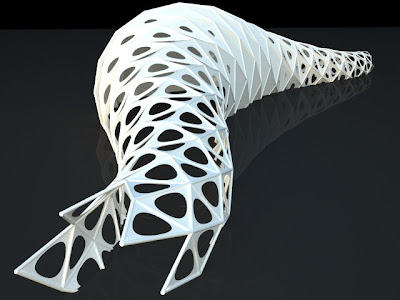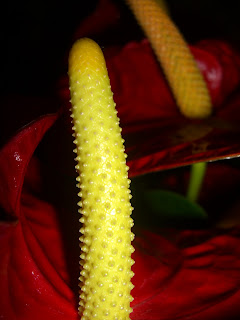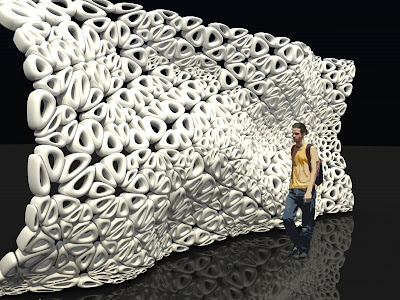## 18.2.09

### phyllotaxis pavilion 01The code (based on the previous phyllotaxis analysis) realizes a pavilion along a selected curve. Pavilion internal radius is influenced by the distance between the curve and an attractor point. Holes-size is related to the distance between the curve and another attractor point (placed in this case near curve's midpoint). In this way holes become smaller moving towards curve's midpoint.

## 8.2.09

### phyllotaxis 01

From wikipedia:

In botany, phyllotaxis is the arrangement of the leaves on the stem of a plant. Phyllotactic architecture optimizes access to moisture, rainfall and sunlight.
The basic patterns are alternate, opposite, whorled or spiral.
The repeating spiral can be represented by a fraction describing the angle of windings leaf per leaf.
Alternate leaves will have an angle of 1/2 of a full rotation. In beech and hazel the angle is 1/3, in oak and apricot it is 2/5, in poplar and pear it is 3/8, and in willow and almond the angle is 5/13. The numerator and denominator normally consist of a Fibonacci number and its second successor. The number of leaves is sometimes called rank, in the case of simple Fibonacci ratios, because the leaves line up in vertical rows. With larger Fibonacci pairs, the pattern becomes complex and non-repeating. This tends to occur with a basal configuration. Examples can be found in composite flowers and seed heads.
The most famous example is the sunflower head. This phyllotactic pattern
creates an optical illusion of criss-crossing spirals. In the botanical literature, these designs are described by the number of counter-clockwise spirals and the number of clockwise spirals. These also turn out to be Fibonacci numbers. In some cases, the numbers appear to be multiples of Fibonacci numbers because the spirals consist of whorls. The florets within the sunflower's cluster are arranged in a spiraling pattern. Typically each floret is oriented toward the next by approximately the golden angle, 137.5°, producing a pattern of interconnecting spirals where the number of left spirals and the number of right spirals are successive Fibonacci numbers. Typically, there are 34 spirals in one direction and 55 in the other; on a very large sunflower there could be 89 in one direction and 144 in the other.I created a script based on Fibonacci spiral. Spiral are created along a curve selected by the user. Radius of the tube-shaped surface on which are settled spirals is influenced from the distance between curve and a selected point.This is my first attempt...I’m working to improve it!!I've seen on opensystem blog an interesting script that uses squares to tessellate a surface.So i created a similar script that uses triangles instead of squares. It's a little bit more complicated because it creates lofted surface in the end.